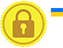# Condition in switch statement

The switch case statement in JavaScript is used for decision making purposes.

In some cases, using the switch case statement is seen to be more convenient over if-else statements.

Consider a situation, when you want check the mark and display the message accordingly.

• If mark is less than or equal to 35 then, fail
• If mark is less greater than or equal to 36 and less than or equal to 60, then Just pass.
• If marks is greater than or equal to 61 and less than or equal to 80 , then Good.
• If mark is greater than equal to 81, then Excellent.

Here the mark is defined as 100 and when switch statement is executed, the condition will be checked as follows.

• case mark <=35
• true => (100 <= 35) - this will give the result false.
• true => false - case 1 will not be executed.
• case mark >=36 && mark <= 60
• true === (100 >=36 && 100 <= 60)
• true === (true && false) - this will give the result false.
• true === false - case 2 will not be executed.
• case mark >= 61 && mark <= 80
• true === (100 >=61 && 100 <= 80)
• true === (true && false) - this will give the result false
• true === false - case 3 will not be executed.
• case mark >= 81
• true === (100 >= 81) - this will give the result false.
• true === (true) - case 4 will be executed.

This is not recommended

### Example

Try Yourself